# Time signature facts for kids

Kids Encyclopedia Facts

A time signature is a set of two numbers, one on top of the other one, written right after the key signature in a piece of music. The two numbers in a time signature tell you how many of one kind of note there are in each measure in the song. For example,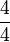$\frac 4 4$ means that there are four beats in each measure and the quarter note gets one beat.

## Finding out what certain time signatures mean

The number in the top of the time signature tells a player how many of a certain kind of note there are in each measure. The number in the bottom of the time signature tells what kind of note is used a certain number of times in each measure. The number on the bottom of the time signature can be any exponent of 2. So, 64 could be a number that is put in the bottom of the time signature, but 65 could not be one.

Number on the bottom of the time signature What it means
1 A whole note lasts one beat
2 A half note lasts one beat
4 A quarter note lasts one beat
8 An eighth note lasts one beat
16 A sixteenth note lasts one beat

This table shows different numbers that could be the bottom of a time signature, and what they mean. Note that each note down lasts for one-half of the amount the note above it lasted. For example, one quarter note lasts one beat, and one-half note lasts one half of a beat, because one divided by two is one-half.

## Time signatures that are used very often

Time signature What it means$\frac 4 4$ Four quarter notes in each measure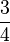$\frac 3 4$ Three quarter notes in each measure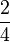$\frac 2 4$ Two quarter notes in each measure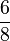$\frac 6 8$ Six eighth notes in each measure

## Symbols that are used instead of time signatures

• The letter C has been used instead of using$\frac 4 4$ time.
• The symbol "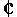" is called "cut time" (alla breve) and has been used instead of using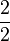$\frac 2 2$ time, where every note is cut in half. So, in "cut time", a quarter note, which usually gets one beat, gets one-half of a beat.

## Images for kidsTime signature Facts for Kids. Kiddle Encyclopedia.